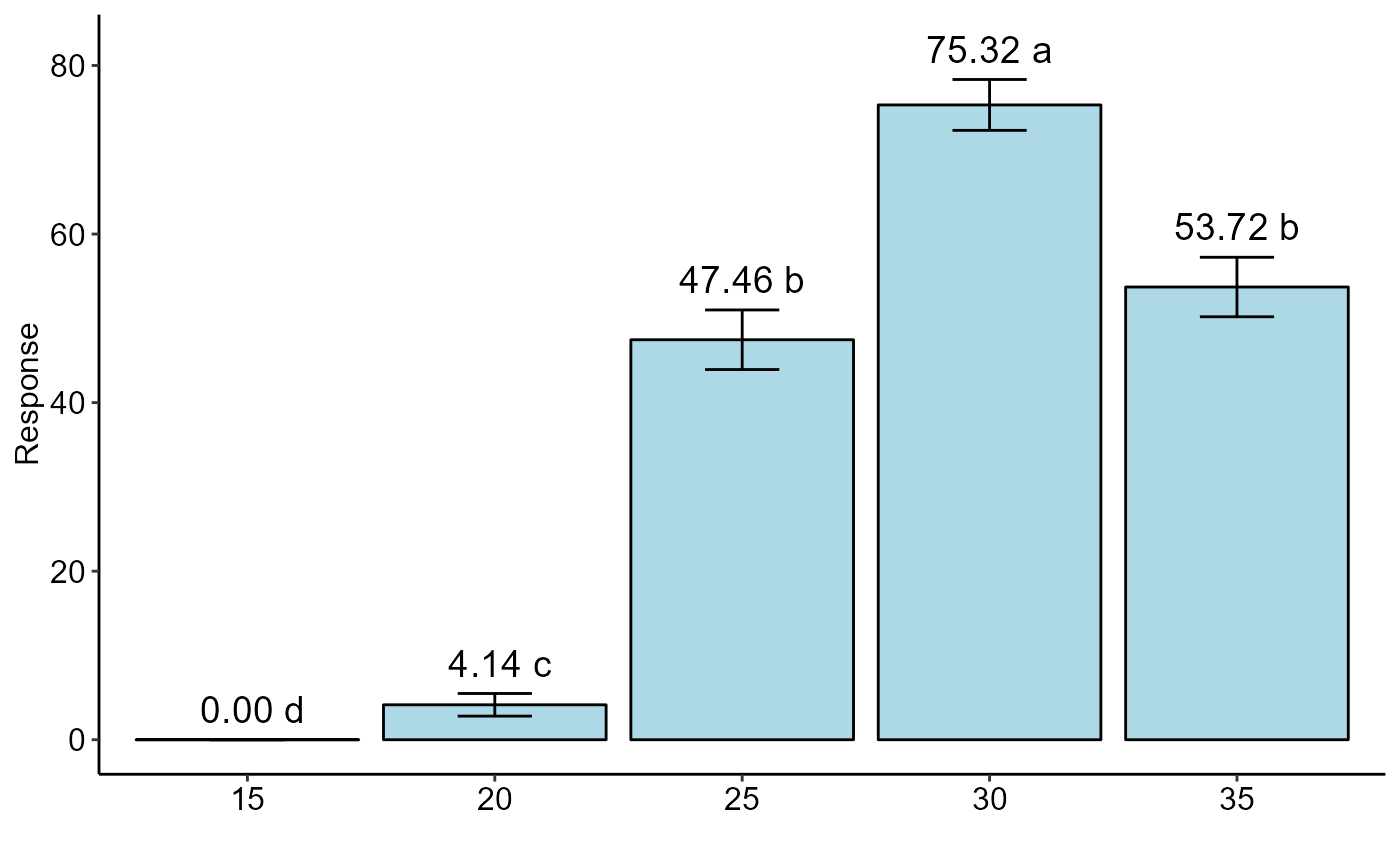Statistical analysis of experiments conducted in a randomized block design using a generalized linear model. It performs the deviance analysis and the effect is tested by a chi-square test. Multiple comparisons are adjusted by Tukey.

DBC.glm(
trat,
block,
response,
glm.family = "binomial",
quali = TRUE,
alpha.f = 0.05,
alpha.t = 0.05,
geom = "bar",
theme = theme_classic(),
sup = NA,
ylab = "Response",
xlab = "",
fill = "lightblue",
angle = 0,
family = "sans",
textsize = 12,
labelsize = 5,
dec = 3,
errorbar = TRUE,
posi = "top",
point = "mean_sd",
angle.label = 0
)

## Arguments

trat

Numerical or complex vector with treatments

block

Numerical or complex vector with blocks

response

Numerical vector containing the response of the experiment. Use cbind(resp, n-resp) for binomial or quasibinomial family.

glm.family

distribution family considered (default is binomial)

quali

Defines whether the factor is quantitative or qualitative (default is qualitative)

alpha.f

Level of significance of the F test (default is 0.05)

alpha.t

Significance level of the multiple comparison test (default is 0.05)

geom

Graph type (columns, boxes or segments)

theme

ggplot2 theme (default is theme_classic())

sup

Number of units above the standard deviation or average bar on the graph

ylab

Variable response name (Accepts the expression() function)

xlab

Treatments name (Accepts the expression() function)

fill

Defines chart color (to generate different colors for different treatments, define fill = "trat")

angle

x-axis scale text rotation

family

Font family

textsize

Font size

labelsize

Label size

dec

Number of cells

Plot the average value on the graph (default is TRUE)

errorbar

Plot the standard deviation bar on the graph (In the case of a segment and column graph) - default is TRUE

posi

Legend position

point

Defines whether to plot mean ("mean"), mean with standard deviation ("mean_sd" - default) or mean with standard error (default - "mean_se").

angle.label

label angle

## Author

Gabriel Danilo Shimizu, shimizu@uel.br

Leandro Simoes Azeredo Goncalves

Rodrigo Yudi Palhaci Marubayashi

## Examples

data("aristolochia")
attach(aristolochia)
#> The following object is masked from cloro:
#>
#>     resp
#> The following object is masked from passiflora:
#>
#>     trat
# Assuming the same aristolochia data set, but considering randomized blocks
bloco=rep(paste("B",1:16),5)
resp=resp/2
DBC.glm(trat,bloco, cbind(resp,50-resp), glm.family="binomial")
#>
#>
#> -----------------------------------------------------------------
#> Analysis of deviance
#> -----------------------------------------------------------------
#>
#> Null deviance        2159.834
#> Df Null deviance       79.000
#> -----
#> Treatment effects
#> Residual deviance     346.688
#> Df residual deviance   75.000
#> p-value(Chisq)          0.000
#> -----
#> Block effects
#> Residual deviance     247.739
#> Df residual deviance   60.000
#> p-value(Chisq)          0.000
#> -----
#> AIC                   510.227
#>
#>
#> As the calculated p-value, it is less than the 5% significance level.The hypothesis H0 of equality of means is rejected. Therefore, at least two treatments differ
#>
#>
#> -----------------------------------------------------------------
#> Multiple Comparison Test
#> -----------------------------------------------------------------
#>    trat prob   SE asymp.LCL asymp.UCL .group
#> 15   15 0.00 0.00      0.00      0.00      d
#> 20   20 0.04 0.01      0.03      0.05     c
#> 25   25 0.47 0.02      0.44      0.51    b
#> 30   30 0.75 0.02      0.72      0.78   a
#> 35   35 0.54 0.02      0.50      0.57    b